# RS Aggarwal Solutions Chapter 12 Circles Exercise 12B Class 10 MathsChapter Name RS Aggarwal Chapter 12 Circles Book Name RS Aggarwal Mathematics for Class 10 Other Exercises Exercise 12AMCQ Exercise Related Study NCERT Solutions for Class 10 Maths

### Exercise 12B Solutions

1. In the adjoining figure, a circle touches all the four sides of a quadrilateral ABCD whose sides are AB = 6 cm, BC = 9 cm and CD = 8 cm. Find the length of side AD.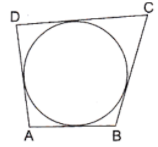Solution

We know that when a quadrilateral circumscribes a circle then sum of opposite sides is equal to the sum of other opposite sides.

∴ AB + CD = AD + BC

⇒ 6 + 8 = AD = 9

2. In the given figure, PA and PB are two tangents to the circle with centre O. If APB = 50° then what is the measure of OAB.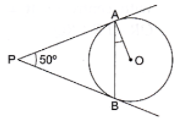Solution

Construction: Join OB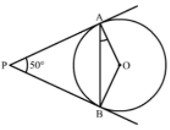We know that the radius and tangent are perpendicular at their point of contact

∵ ∠OBP = ∠OAP = 90°

∠AOB + ∠OBP + ∠APB + ∠OAP = 360° [Angle sum property of a quadrilateral]

⇒ ∠AOB + 90° + 50° + 90° = 360°

⇒ 230° + ∠BOC = 360°

⇒ ∠AOB = 130°

Now, In isosceles triangle AOB

∠AOB + ∠OAB + ∠OBA = 180° [Angle sum property of a triangle]

⇒ 130° + 2∠OAB = 180° [∵ ∠OAB = ∠OBA]

⇒ ∠OAB = 25°

3. In the given figure, O is the centre of a circle. PT and PQ are tangents to the circle from an external point P. If TPQ = 70°, find the TRQ.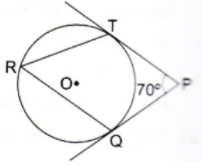Solution

Construction: Join OQ and OT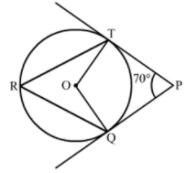We know that the radius and tangent are perpendicular at their point of contact

∵ ∠OTP = ∠OQP = 90°

∠QOT + ∠OTP + ∠OQP + ∠TPO = 360° [Angle sum property of a quadrilateral]

⇒ ∠QOT + 90° + 90° = 70° = 360°

⇒ 250° + ∠QOT = 360°

⇒ ∠QOT = 110°

We know that the angle subtended by an arc at the center is double the angle subtended by the arc at any point on the remaining part of the circle.

∴ ∠TRQ = 1/2(∠QOT) = 55°

4. In the given figure common tangents AB and CD to the two circles with centres O1 and O2 intersect at E. Prove that AB = CD.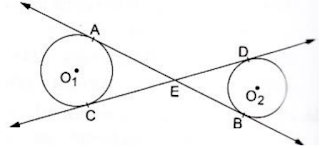Solution

We know that tangent segments to a circle from the same external point are congruent.

So, we have

EA = EC for the circle having center O1

and

ED = EB for the circle having centre O1

Now, Adding ED on both sides in EA = EC, we get

EA + ED = EC + ED

⇒ EA + EB = EC + ED

⇒ AB = CD

5. If PT is a tangent to a circle with center O and PQ is a chord of the circle such that ∠QPT  = 70°, then find the measure of POQ.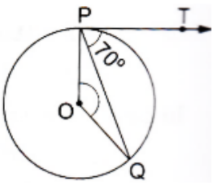Solution

We know that the radius and tangent are perpendicular at their point of contact.

∴ ∠OPT = 90°

Now, ∠OPQ = ∠OPT - ∠TPQ = 90° - 70° = 20°

Since, OP = OQ as both are radius

∴ ∠OPQ = ∠OQP = 20° (Angles opposite to equal sides are equal)

Now,

In isosceles ∆POQ

∠POQ + OPQ + OQP = 180° (Angle sum property of a triangle)

⇒ ∠POQ = 180° - 20° = 140°

6. In the given figure, a triangle ABC is drawn to circumscribe a circle of radius 2 cm such that the segments BD and DC into which BC is divided by the point of contact D, are of lengths 4 cm and 3 cm respectively. If the area of ∆ABC = 21 cm2 then find the lengths of sides AB and AC.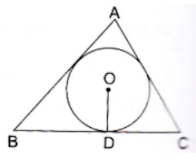Solution

Construction: Join OA, OB, OC, OE ⊥ AB at E and OF ⊥ AC at F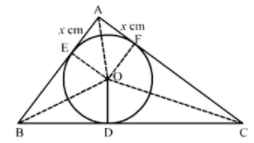We know that tangent segments to a circle from the same external point are congruent

Now, we have

AE = AF, BD = BE = 4 cm and CD = CF = 3 cm

Now,

Area (ABC) = Area(∆BOC) + Area(∆AOB) + Area (AOC)

⇒ 21 = 1/2 × BC × OD + 1/2 × AB × OE + 1/2 × AC × OF

⇒ 42 = 7 × 2 + (4 + x) × 2 + (3 + x) × 2

⇒ 21 = 7 + 4 + x + 3 + x

⇒ 21 = 14 + 2x

⇒ 2x = 7

⇒ x = 3.5 cm

∴ AB = 4 + 3.5 = 7.5 cm and AC = 3 + 3.5 = 6.5 cm

7. Two concentric circles are of radii5 cm and 3 cm. Find the length of the chord of the larger circle (in cm) which touches the smaller circle.

Solution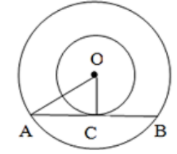Given two circles have the same center O and AB is a chord of the larger circle touching the smaller circle at C; also, OA = 5 cm and OC = 3 cm

In ∆OAC, OA2 = OC2 + AC2

∴ AC2 = OA2 – OC2

⇒ AC2 = 52 – 32

⇒ AC2 = 25 – 9

⇒ AC2 = 16

⇒ AC = 4 cm

∴ AB = 2AC (Since perpendicular drawn from the center of the circle bisects the chord)

∴ AB = 2 × 4 = 8 cm

The length of the chord of the larger circle is 8 cm.

8. Prove that the perpendicular at the point of contact of the tangent to a circle passes through the centre.

Solution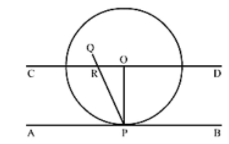Let AB be the tangent to the circle at point P with center O.

To prove: PQ passes through the point O.

Construction: Join OP

Through O, draw a straight line CD parallel to the tangent AB.

Proof: Suppose that PQ doesn’t passes through point O.

PQ intersect CD at R and also intersect AB at P.

AS, CD ∥ AB. PQ is the line of intersection.

∠ORP = ∠RPA (Alternate interior angles) but also.

∠RPA = 90° (OP ⊥ AB)

⇒ ∠ORP = 90°

∠ROP + ∠OPA = 180° (Co-interior angles)

⇒ ∠ROP + 90° = 180°

⇒ ∠ROP = 90°

Thus, the ∆ORP has 2 right angles i.e., ∠ORP and ∠ROP which is not possible

Hence, our supposition is wrong

∴ PQ passes through the point O.

9. In the given figure, two tangents RQ, and RP and RP are drawn from an external point R to the circle with centre O. If PRQ = 120°, then prove that OR = PR + RQ.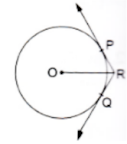Solution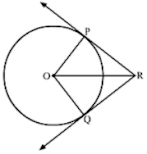Construction Join PO and OQ

In ∆POR and ∆QOR

RP = RQ (Tangents from the external point are congruent).

OR = OR (Common)

By SSS congruency, ∆POR ∆QOR

∠PRO = ∠QRO (C.P.C.T)

Now, ∠PRO + ∠QRO = ∠PRQ

⇒ 2∠PRO = 120°

⇒ ∠PRO = 60°

Now, In ∆POR

cos 60° = PR/OR

⇒ 1/2 = PR/OR

⇒ OR = 2PR

⇒ OR = PR + PR

⇒ OR = PR + RQ

10. In the given figure, a cradle inscribed in a triangle ABC touches the sides AB, BC and CA at points D, E and F respectively. If AB = 14 cm, BC = 8 cm and CA = 12 cm. Find the length AD, BE and CF.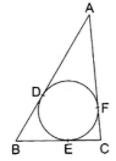Solution

We know that segments to a circle from the same external point are congruent

Now, we have

AD = AF, BD = BE and CE = CF

Now, AD + BD = 14 cm ....(1)

AF + FC = 12 cm

⇒ AD + FC = 12 cm ...(2)

BE + EC = 8 cm

⇒ BD + FC = 8 cm ...(3)

AD + BD + AD + FC + BD + FC = 342

⇒ 2(AD + BD + FC) = 34

⇒ AD + BO + FC = 17 cm ...(4)

Solving (1) and (4), we get

FC = 3 cm

Solving (2) and (4), we get

BD = 5 cm = BE

Solving (3) and (4), we get

11. In the given figure, O is the centre of the circle, PA and PB are tangents. Show that AOBP is cyclic quadrilateral.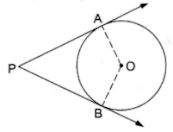Solution

We know that the radius and tangent are perpendicular at their point of contact

∵ ∠OBP = ∠OAP = 90°

∠APB + ∠AOB + ∠OBP + ∠OAP = 360° [Angle sum property of a quadrilateral]

⇒ ∠APB + ∠AOB + 90° + 90° = 360°

⇒ ∠APB + ∠AOB = 180°

Since, the sum of the opposite angles of the quadrilateral is 180°

Hence, AOBP is a cyclic quadrilateral.

12. In two concentric circles. a chord of length 8 cm of the large circle touches the smaller circle. If the radius of the larger circle is 5 cm then find the radius of the smaller circle.

Solution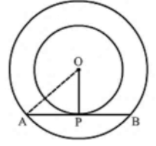We know that the radius and tangent are perpendicular at their point of contact

Since, the perpendicular drawn from the centre bisect the chord

∴ AP = PB = AB/2 = 4 cm

In right triangle AOP

AO2 = OP2 + PA2

⇒ 52 = OP2 + 42

⇒ OP2 = 9

⇒ OP = 3 cm

Hence, the radius of the smaller circle is 3 cm.

13. In the given figure, PQ is chord of a circle with centre O an PT is a tangent. If QPT = 60°, find the PRQ.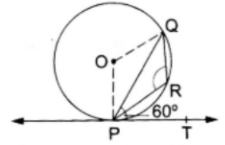Solution

We know that the radius and tangent are perpendicular at their point of contact

∴ ∠OPT = 90°

Now, ∠OPQ = ∠OPT - ∠QPT = 90° - 60° = 30°

Since, OP = OQ as born is radius

∴ ∠OPQ = ∠OQP = 30° (Angles opposite to equal sides are equal)

Now, In isosceles, POQ

∠POQ + ∠OPQ + ∠OQP = 180° (Angle sum property of a triangle)

⇒ POQ = 180° - 30° - 30° = 120°

Now, ∠POQ + reflex ∠POQ = 360° (Complete angle)

⇒ reflex ∠POQ = 360° - 120° = 240°

We know that the angle subtended by an arc at the centre double the angle subtended by the arc at any point on the remaining part of the circle.

∴ ∠PRQ = 1/2 (reflect ∠POQ) = 120°

14. In the given figure, PA and PB are two tangents to the circle with centre O. If APB = 60°, then find the measure of AOB.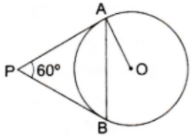Solution

Construction: Join OB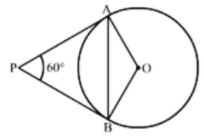We know that the radius and tangent are perpendicular at their point of contact

∵ ∠OBP = ∠OAP = 90°

∠AOB + ∠OBP + ∠APB + ∠OAP = 360° [Angle sum property of a quadrilateral]

⇒ ∠AOB + 90° + 60° + 90° = 360°

⇒ 240° + ∠AOB = 360°

⇒ ∠AOB + 90° + 60° + 90° = 360°

⇒ 240° + ∠AOB = 360°

⇒ ∠AOB = 120°

Now, In isosceles triangle AOB

∠AOB + ∠OAB + ∠OBA = 180° [Angle sum property of a triangle]

⇒ 120° + 2∠OAB = 180° [∵ ∠OAB = ∠OBA]

⇒ ∠OAB = 30°